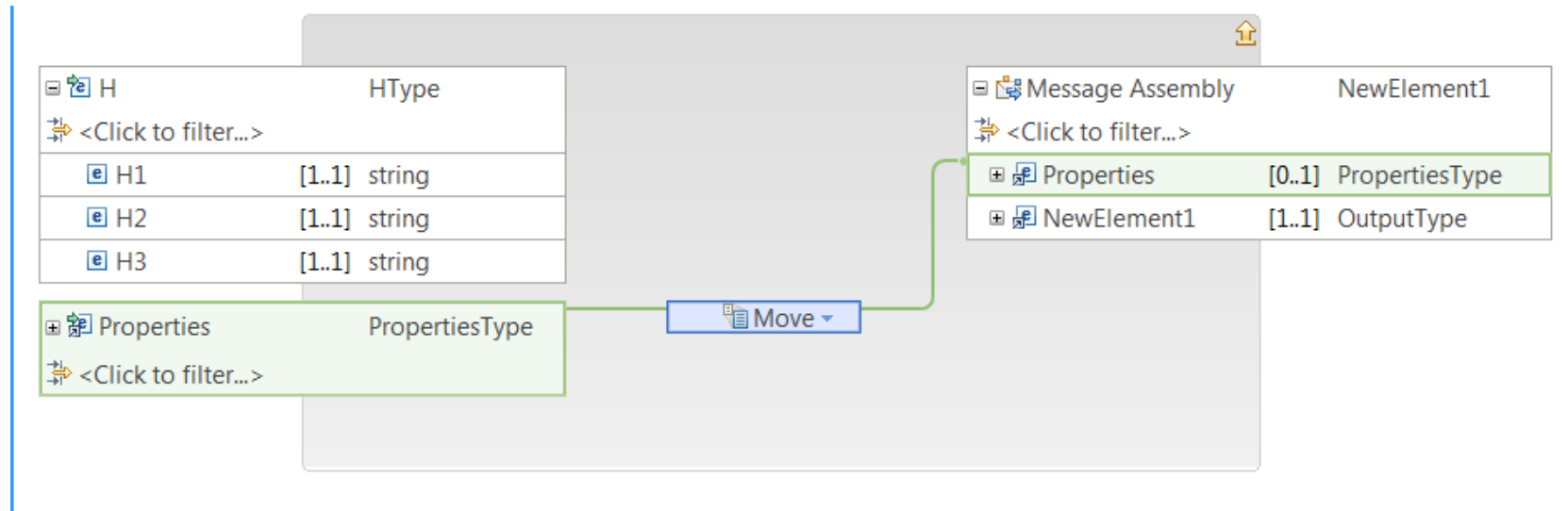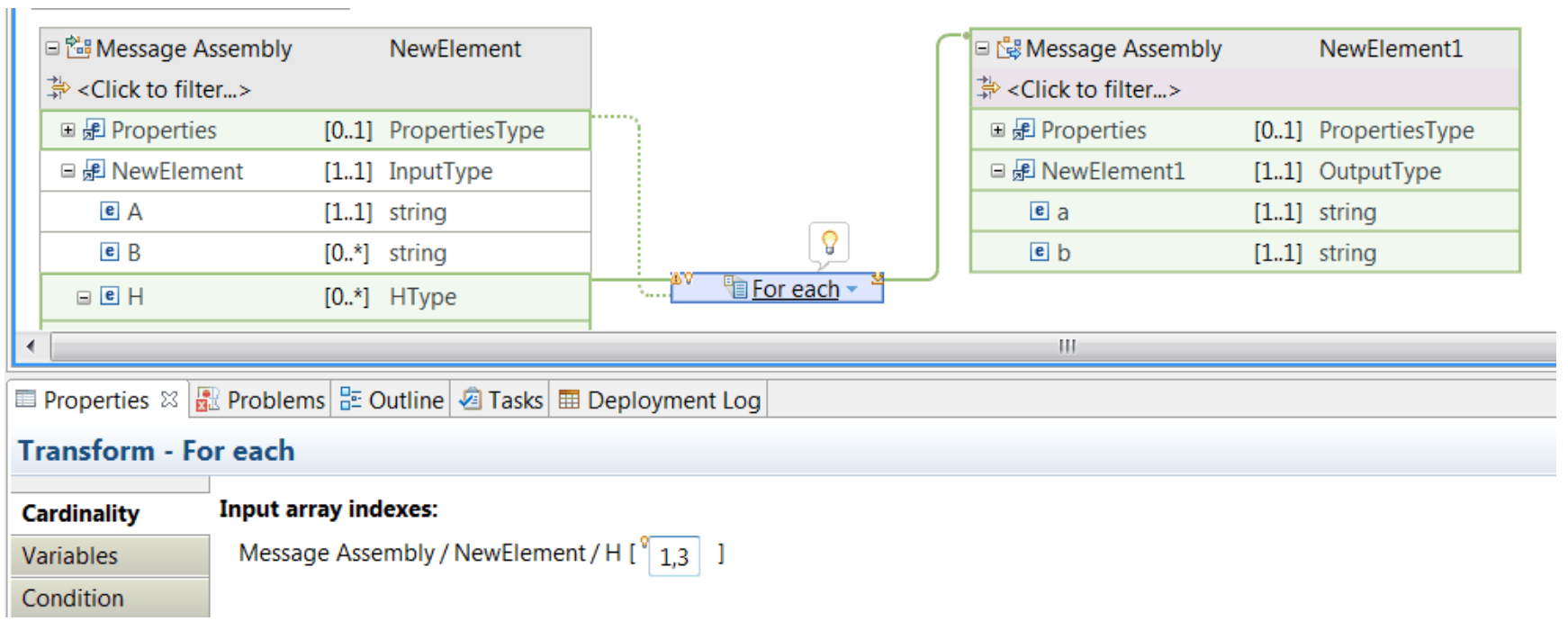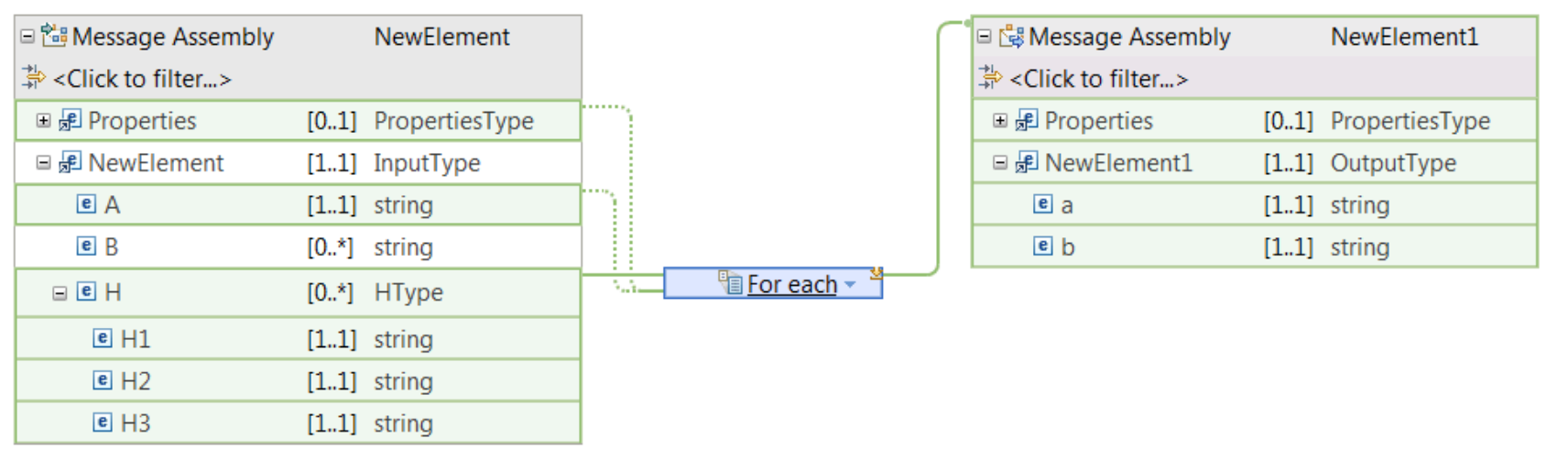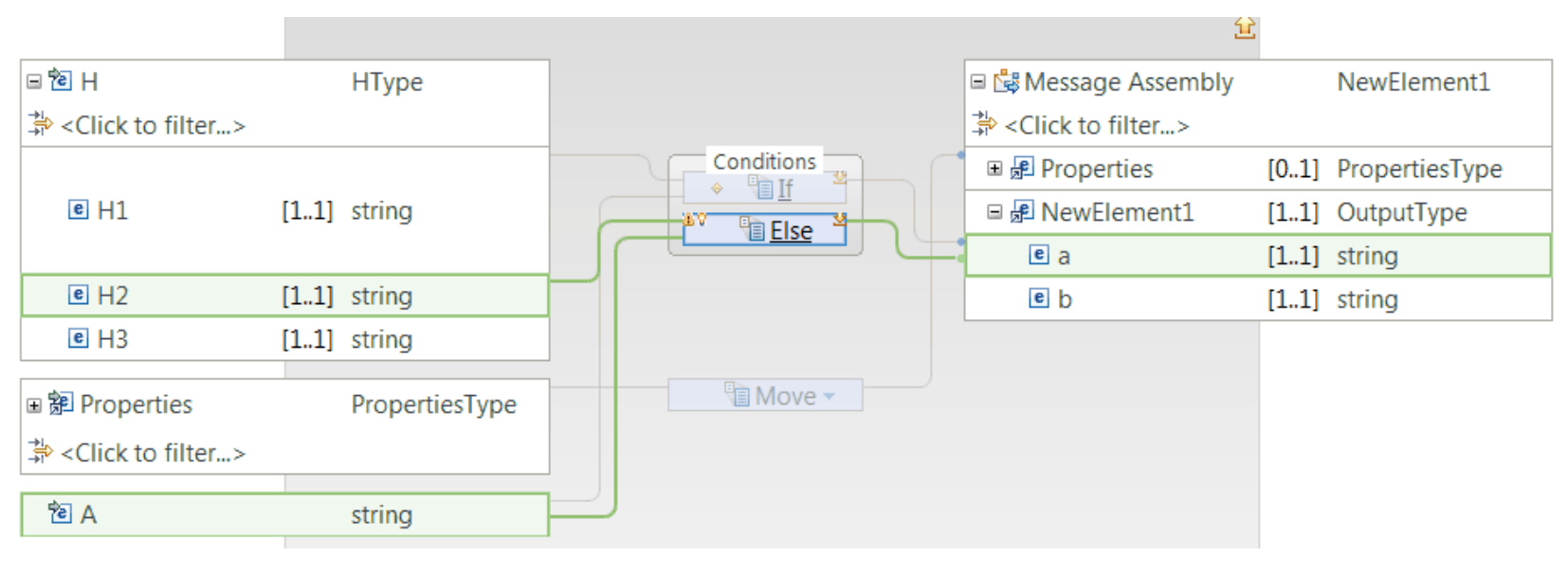# App Connect

View Only

## How to split a message into multiple output messages in the Graphical Mapping editor of IBM Integration Bus

Tue June 02, 2020 09:25 AM

In IBM Integration Bus, you can use the Graphical Data Mapping editor to split an input message into multiple output messages.

You can use a For Each transform or a Join transform wired to the head of the output message assembly to create a map that takes a single input message and produces multiple instances of an output message model. A typical use of this function is message splitting, in which an input batch message is divided into individual record messages.When the map runs, a new message is propagated for each iteration of the For Each or Join transform.

The following steps show you how to split a message:

1. Create a map, and add one input element to your input message assembly, and one output element to your output message assembly.

The input message assembly must contain a repeating element. The values of the elements for each index are used to populate each output message instance.2. Define a “For Each” transform between the input element and the head of the output message assembly.The Move transform between the input and the output Properties tree will display an error. Continue with the steps to remove the error.

3. Delete the Move transform, and then connect with a supplement connection the input Properties tree to the For Each transform.4. Select the For Each transform to open the nested map. Then, define a Move transform between the input and the output Properties tree.5. Optional: Return to the For Each transform, and configure the indexes of the For Each transform for which you want to generate an output message.

If you need to create an output message for each index of the repeating structure, then continue with the next step.You can configure the Input array indexes section to select specific instances of the input array.

6. Optional: If you need additional information from the input message to populate output elements, define a supplement connection between each input element to the For Each transform.

When you connect additional input elements with supplement connections to a For Each transform, you can use these elements as part of your mapping operations within the nested map.7. Select the For Each transform to open the nested map. Then, define the transformation logic inside the For Each nested map.
1. Open the For Each transform nested map.
2. Define an If transform between H and a.
• Define a primary connection between H and the If transform.
• Define a supplement connection between A and the If transform.
• Define a connection between the If transform and the output element a.3. Open the nested map associated to the If transform. Then, define a Move transform between H1 and a. This operation defines the transformation logic that the If transform performs.• Define a primary connection between H and the Else transform.
• Define a supplement connection between A and the Else transform.
• Define a connection between the Else transform and the output element a.5. Open the nested map associated with the Else transform, and then define a Move transform between H2 and a. This operation defines the transformation logic that the Else transform performs.### Result

The following figure shows the For Each transform nested map after all the transformation logic has been implemented:#IBMIntegrationBus
#IIB
#GDM